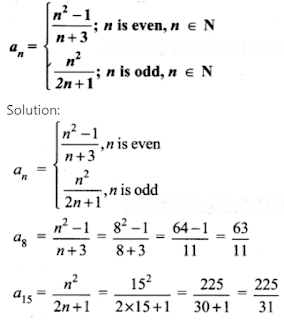Welcome to Our Tamil Crowd Website..You Will Get All Job news, Tamil breaking news, Educational news, Health Tips here..

### 10th Maths- Chapter 2-  Numbers and Sequences Exercise  2.4 (All Sums)

Question 1.

Find the next three terms of the following sequence.

(i) 8, 24, 72, …….

(ii) 5, 1, -3, …….

(iii) 14,29,316………..

Solution:

(i) 8, 24, 72…

In an arithmetic sequence a = 8,

d = t1 – t1 = t3 – t2

= 24 – 8       72 – 24

= 16 ≠ 48

So, it is not an arithmetic sequence. In a geometric sequence,

r = t2t1=t3t2

⇒ 248=7224

⇒ 3 = 3

∴ It is a geometric sequence

∴ The nth term of a G.P is tn = arn-1

∴ t4 = 8 × 34-1

= 8 × 33

= 8 × 27

= 216

t5 = 8 × 35-1

= 8 × 34

= 8 × 81

= 648

t6 = 8 × 36-1

= 8 × 35

= 8 × 243

= 1944

The next 3 terms are 8, 24, 72, 216, 648, 1944.

(ii) 5, 1, -3, …

d = t2 – t1 = t3 – t2

⇒ 1 – 5 = -3-1

-4 = -4 ∴ It is an A.P.

tn = a+(n – 1)d

t4 = 5 + 3 × – 4

= 5 – 12

= -7

15 = a + 4d

= 5 + 4 × -4

= 5 – 16

= -11

t6 = a + 5d

= 5 + 5 × – 4

= 5 – 20

= – 15

∴ The next three terms are 5, 1, -3, -7, -11, -15.

(iii) 14,29,316,………..

Here an = Numerators are natural numbers and denominators are squares of the next numbers

14,29,316,425,536,649………….

Question 2.

Find the first four terms of the sequences whose nth terms are given by

(i) an = n3 -2

Solution:

an = n3 – 2

a1 = 13 – 2 = 1 – 2 = -1

a2 = 23 – 2 = 8 – 2 = 6

a3 = 33 – 2 = 27 – 2 = 25

a4 = 43 – 2 = 64 – 2 = 62

The four terms are -1, 6, 25 and 62

(ii) an = (-1)n+1 n(n + 1)

Solution:

an = (-1)n+1 n(n + 1)

a1 = (-1)2 (1) (2) = 1 × 1 × 2 = 2

a2 = (-1)3 (2) (3) = -1 × 2 × 3 = -6

a3 = (-1)4 (3) (4) = 1 × 3 × 4 = 12

a4 = (-1)5 (4) (5) = -1 × 4 × 5 = -20

The four terms are 2, -6, 12 and -20

(iii) an = 2n2 – 6

Solution:

an = 2 n2 – 6

a1 = 2(1)2 – 6 = 2 – 6 = -4

a2 = 2(2)2 – 6 = 8 – 6 = 2

a3 = 2(3)2 – 6 = 18 – 6 = 12

a4 = 2(4)2 – 6 = 32 – 6 = 26

The four terms are -4, 2, 12, 26

Question 3.

Find the nth term of the following sequences

(i) 2, 5, 10, 17, ……….

(ii) 0, 12, 23,…..

(iii) 3, 8, 13, 18, ………

Solution:

(i) 2, 5, 10, 17

= 12 + 1, 22 + 1, 32 + 1, 42 + 1 ……….

∴ nth term is n2+1

(ii) 0, 12,23,………….

= 1−11,2−12,3−13…..

⇒ n−1n

∴ nth term is n−1n

(iii) 3, 8, 13, 18

a = 3

d = 5

tn = a + (n – 1)d

= 3 + (n – 1)5

= 3 + 5n – 5

= 5n – 2

∴ nth term is 5n – 2

Question 4.

Find the indicated terms of the sequences whose nth terms are given by

(i) an = 5nn+2 ; a6 and a13

(ii) an = -(n2 – 4); a4 and a11

Solution:

(i) an = 5n/(n + 2)

a6 = 5(6)/(6 + 2) = 30/8 = 15/4

a13 = 5(13)/(13 + 2) = (5 x 13)/15 = 13/3

a6 = 15/4, a13 = 13/3

(ii) an = -(n2 – 4)

a4 = -(42 – 4)

= – (16 – 4)

= -12

a11 = -(112 – 4)

= – (121 – 4)

= – 117

a4 = -12 and a11 = -117

Question 5.

Find a8 and a15 whose nth term isQuestion 6.

If a1 = 1, a2 = 1 and an = 2an-1 + an-2 n > 3, n ∈ N. Then find the first six terms of the sequence.

Solution:

a1 = a2 = 1

an = 2an-1 + an-2

a3 = 2a3-1 + a3-2 = 2a2 + a1

= 2(1) + 1 = 3

a4 = 2a4-1 + a4-2

= 2a3 + a2

= 2(3) + 1 = 6 + 1 = 7

a5 = 2 a5-1 + a5-2

= 2a4 + a3

= 2(7) + 3 = 17

a6 = 2a6-1 + a6-2

= 2a5 + a4

= 2(17) + 7

= 34 + 7 = 41

The sequence is 1, 1, 3, 7, 17,41, …